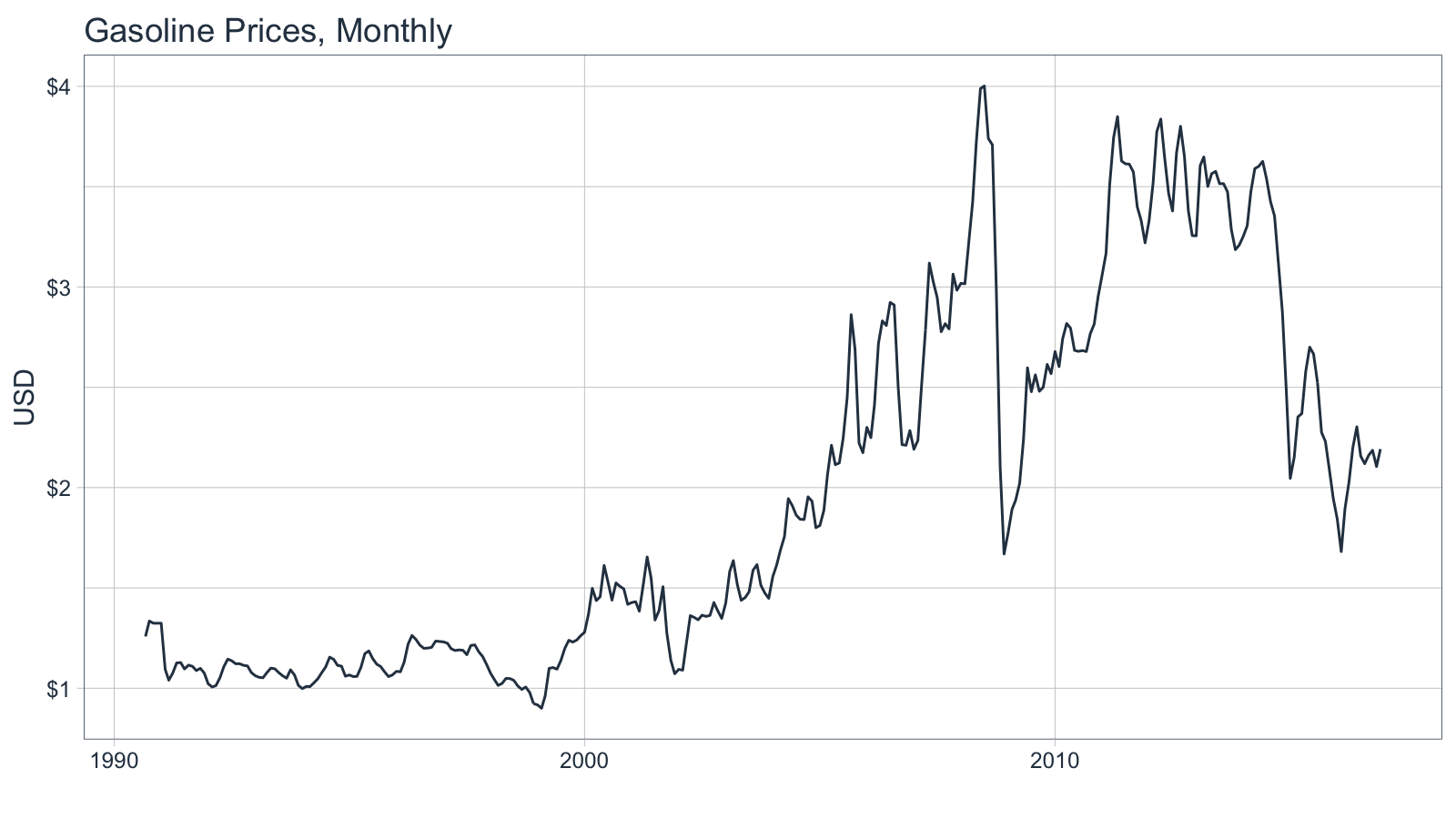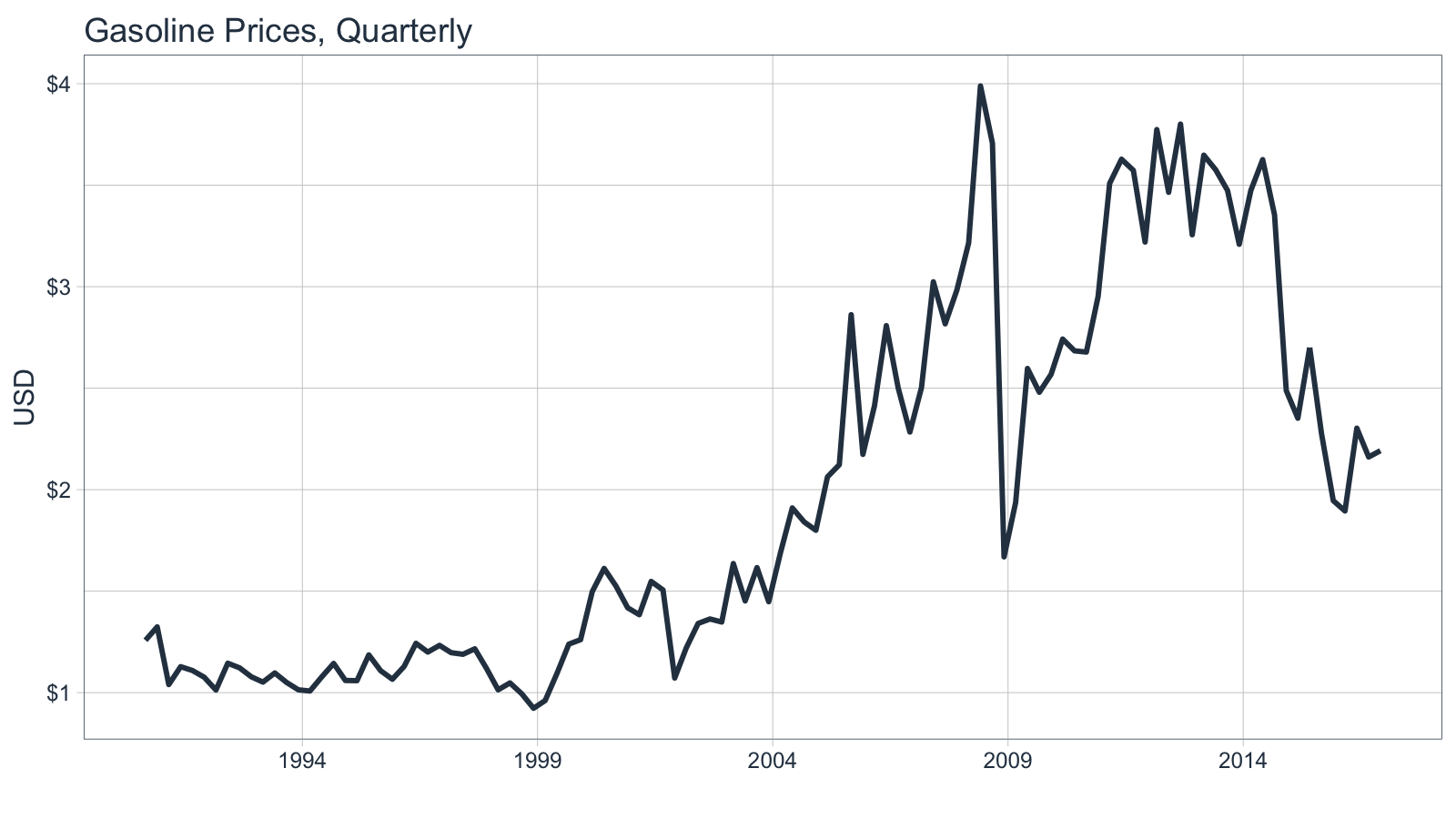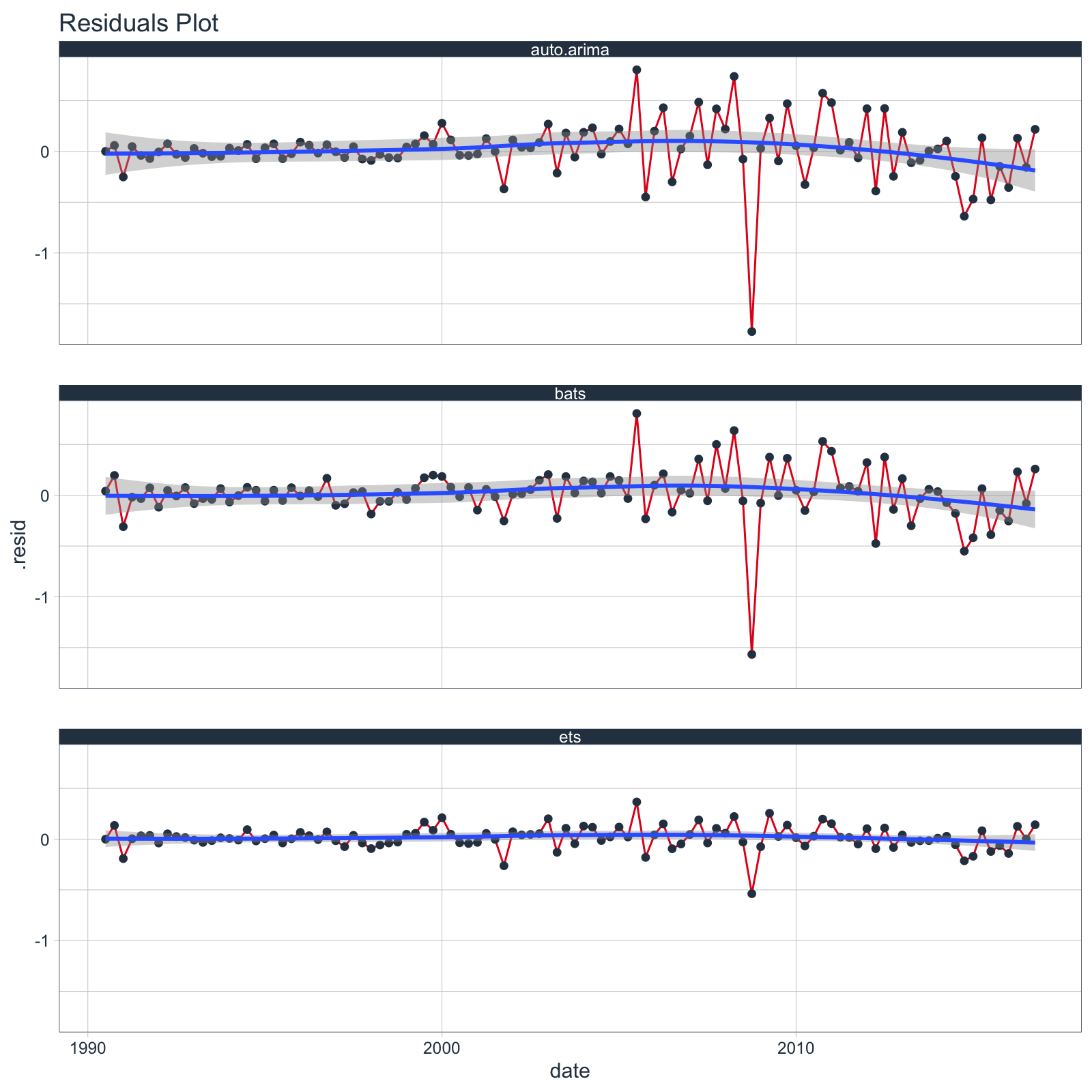# Forecasting Using Multiple Models

#### 2019-10-08

Extending `broom` to time series forecasting

One of the most powerful benefits of `sweep` is that it helps forecasting at scale within the “tidyverse”. There are two common situations:

1. Applying a model to groups of time series
2. Applying multiple models to a time series

In this vignette we’ll review how `sweep` can help the second situation: Applying multiple models to a time series.

# Prerequisites

Before we get started, load the following packages.

``````library(tidyverse)
library(tidyquant)
library(timetk)
library(sweep)
library(forecast)``````

# Forecasting Gasoline Prices

To start, let’s get some data from the FRED data base using `tidyquant`. We’ll use `tq_get()` to retrieve the Gasoline Prices from 1990 through today (2019-10-08).

``````gas_prices_monthly_raw <- tq_get(
x    = "GASREGCOVM",
get  = "economic.data",
from = "1990-01-01",
to   = "2016-12-31")
gas_prices_monthly_raw``````
``````## # A tibble: 316 x 2
##    date       price
##    <date>     <dbl>
##  1 1990-09-01  1.26
##  2 1990-10-01  1.34
##  3 1990-11-01  1.32
##  4 1990-12-01 NA
##  5 1991-01-01 NA
##  6 1991-02-01  1.09
##  7 1991-03-01  1.04
##  8 1991-04-01  1.08
##  9 1991-05-01  1.13
## 10 1991-06-01  1.13
## # … with 306 more rows``````

Upon a brief inspection, the data contains 2 `NA` values that will need to be dealt with.

``summary(gas_prices_monthly_raw\$price)``
``````##    Min. 1st Qu.  Median    Mean 3rd Qu.    Max.    NA's
##   0.900   1.138   1.615   1.974   2.697   4.002       2``````

We can use the `fill()` from the `tidyr` package to help deal with these data. We first fill down and then fill up to use the previous and then post days prices to fill in the missing data.

``````gas_prices_monthly <- gas_prices_monthly_raw %>%
fill(price, .direction = "down") %>%
fill(price, .direction = "up")``````

We can now visualize the data.

``````gas_prices_monthly %>%
ggplot(aes(x = date, y = price)) +
geom_line(color = palette_light()[]) +
labs(title = "Gasoline Prices, Monthly", x = "", y = "USD") +
scale_y_continuous(labels = scales::dollar) +
theme_tq()``````Monthly periodicity might be a bit granular for model fitting. We can easily switch periodicity to quarterly using `tq_transmute()` from the `tidyquant` package along with the periodicity aggregation function `to.period` from the `xts` package. We’ll convert the date to `yearqtr` class which is regularized.

``````gas_prices_quarterly <- gas_prices_monthly %>%
tq_transmute(mutate_fun = to.period, period = "quarters")
gas_prices_quarterly``````
``````## # A tibble: 106 x 2
##    date       price
##    <date>     <dbl>
##  1 1990-09-01  1.26
##  2 1990-12-01  1.32
##  3 1991-03-01  1.04
##  4 1991-06-01  1.13
##  5 1991-09-01  1.11
##  6 1991-12-01  1.08
##  7 1992-03-01  1.01
##  8 1992-06-01  1.14
##  9 1992-09-01  1.12
## 10 1992-12-01  1.08
## # … with 96 more rows``````

Another quick visualization to show the reduction in granularity.

``````gas_prices_quarterly %>%
ggplot(aes(x = date, y = price)) +
geom_line(color = palette_light()[], size = 1) +
labs(title = "Gasoline Prices, Quarterly", x = "", y = "USD") +
scale_y_continuous(labels = scales::dollar) +
scale_x_date(date_breaks = "5 years", date_labels = "%Y") +
theme_tq()``````# Performing Forecasts Using Multiple Models

In this section we will use three models to forecast gasoline prices:

1. ARIMA
2. ETS
3. BATS

## Multiple Models Concept

Before we jump into modeling, let’s take a look at the multiple model process from R for Data Science, Chapter 25 Many Models. We first create a data frame from a named list. The example below has two columns: “f” the functions as text, and “params” a nested list of parameters we will pass to the respective function in column “f”.

``````df <- tibble(
f = c("runif", "rpois", "rnorm"),
params = list(
list(n = 10),
list(n = 5, lambda = 10),
list(n = 10, mean = -3, sd = 10)
)
)
df``````
``````## # A tibble: 3 x 2
##   f     params
##   <chr> <list>
## 1 runif <named list >
## 2 rpois <named list >
## 3 rnorm <named list >``````

We can also view the contents of the `df\$params` column to understand the underlying structure. Notice that there are three primary levels and then secondary levels containing the name-value pairs of parameters. This format is important.

``df\$params``
``````## []
## []\$n
##  10
##
##
## []
## []\$n
##  5
##
## []\$lambda
##  10
##
##
## []
## []\$n
##  10
##
## []\$mean
##  -3
##
## []\$sd
##  10``````

Next we apply the functions to the parameters using a special function, `invoke_map()`. The parameter lists in the “params” column are passed to the function in the “f” column. The output is in a nested list-column named “out”.

``````df_out <- df %>%
mutate(out = invoke_map(f, params))
df_out``````
``````## # A tibble: 3 x 3
##   f     params           out
##   <chr> <list>           <list>
## 1 runif <named list > <dbl >
## 2 rpois <named list > <int >
## 3 rnorm <named list > <dbl >``````

And, here’s the contents of “out”, which is the result of mapping a list of functions to a list of parameters. Pretty powerful!

``df_out\$out``
``````## []
##   0.3539214 0.8305328 0.1725492 0.3336279 0.4272752 0.1765578 0.8405683
##   0.1642450 0.8351444 0.5650580
##
## []
##  11  5  9 14  7
##
## []
##    -7.814870 -15.960203  -4.229270  -5.531148 -17.662205  -3.518611
##     5.652761   5.394733   1.527318  13.632480``````

Take a minute to understand the conceptual process of the `invoke_map` function and specifically the parameter setup. Once you are comfortable, we can move on to model implementation.

## Multiple Model Implementation

We’ll need to take the following steps to in an actual forecast model implementation:

1. Coerce the data to time series
2. Build a model list using nested lists
3. Create the the model data frame
4. Invoke a function map

This is easier than it sounds. Let’s start by coercing the univariate time series with `tk_ts()`.

``````gas_prices_quarterly_ts <- gas_prices_quarterly %>%
tk_ts(select = -date, start = c(1990, 3), freq = 4)
gas_prices_quarterly_ts``````
``````##       Qtr1  Qtr2  Qtr3  Qtr4
## 1990             1.258 1.324
## 1991 1.040 1.128 1.109 1.076
## 1992 1.013 1.145 1.122 1.078
## 1993 1.052 1.097 1.050 1.014
## 1994 1.008 1.078 1.144 1.060
## 1995 1.059 1.186 1.108 1.066
## 1996 1.129 1.243 1.200 1.233
## 1997 1.197 1.189 1.216 1.119
## 1998 1.014 1.048 0.994 0.923
## 1999 0.961 1.095 1.239 1.261
## 2000 1.498 1.612 1.525 1.418
## 2001 1.384 1.548 1.506 1.072
## 2002 1.221 1.341 1.363 1.348
## 2003 1.636 1.452 1.616 1.448
## 2004 1.689 1.910 1.841 1.800
## 2005 2.063 2.123 2.862 2.174
## 2006 2.413 2.808 2.501 2.284
## 2007 2.503 3.024 2.817 2.984
## 2008 3.215 3.989 3.709 1.669
## 2009 1.937 2.597 2.480 2.568
## 2010 2.742 2.684 2.678 2.951
## 2011 3.509 3.628 3.573 3.220
## 2012 3.774 3.465 3.801 3.256
## 2013 3.648 3.576 3.474 3.209
## 2014 3.474 3.626 3.354 2.488
## 2015 2.352 2.700 2.275 1.946
## 2016 1.895 2.303 2.161 2.192``````

Next, create a nested list using the function names as the first-level keys (this is important as you’ll see in the next step). Pass the model parameters as name-value pairs in the second level.

``````models_list <- list(
auto.arima = list(
y = gas_prices_quarterly_ts
),
ets = list(
y = gas_prices_quarterly_ts,
damped = TRUE
),
bats = list(
y = gas_prices_quarterly_ts
)
)``````

Now, convert to a data frame using the function, `enframe()` that turns lists into tibbles. Set the arguments `name = "f"` and `value = "params"`. In doing so we get a bonus: the model names are the now convieniently located in column “f”.

``````models_tbl <- enframe(models_list, name = "f", value = "params")
models_tbl``````
``````## # A tibble: 3 x 2
##   f          params
##   <chr>      <list>
## 1 auto.arima <named list >
## 2 ets        <named list >
## 3 bats       <named list >``````

We are ready to invoke the map. Combine `mutate()` with `invoke_map()` as follows. Bada bing, bada boom, we now have models fitted using the parameters we defined previously.

``````models_tbl_fit <- models_tbl %>%
mutate(fit = invoke_map(f, params))
models_tbl_fit``````
``````## # A tibble: 3 x 3
##   f          params           fit
##   <chr>      <list>           <list>
## 1 auto.arima <named list > <fr_ARIMA>
## 2 ets        <named list > <ets>
## 3 bats       <named list > <bats>``````

# Inspecting the Model Fit

It’s a good point to review and understand the model output. We can review the model parameters, accuracy measurements, and the residuals using `sw_tidy()`, `sw_glance()`, and `sw_augment()`.

## sw_tidy

The tidying function returns the model parameters and estimates. We use the combination of `mutate` and `map` to iteratively apply the `sw_tidy()` function as a new column named “tidy”. Then we unnest and spread to review the terms by model function.

``````models_tbl_fit %>%
mutate(tidy = map(fit, sw_tidy)) %>%
unnest(tidy) %>%
spread(key = f, value = estimate)``````
``````## # A tibble: 20 x 6
##    params          fit        term            auto.arima      bats      ets
##    <list>          <list>     <chr>                <dbl>     <dbl>    <dbl>
##  1 <named list [1… <fr_ARIMA> ar1                  0.834  NA       NA
##  2 <named list [1… <fr_ARIMA> ma1                 -0.964  NA       NA
##  3 <named list [1… <fr_ARIMA> sar1                 0.939  NA       NA
##  4 <named list [1… <fr_ARIMA> sma1                -0.776  NA       NA
##  5 <named list [1… <bats>     alpha               NA       5.88e-1 NA
##  6 <named list [1… <bats>     ar.coefficients     NA      NA       NA
##  7 <named list [1… <bats>     beta                NA      NA       NA
##  8 <named list [1… <bats>     damping.parame…     NA      NA       NA
##  9 <named list [1… <bats>     gamma.values        NA      -2.62e-2 NA
## 10 <named list [1… <bats>     lambda              NA       6.05e-5 NA
## 11 <named list [1… <bats>     ma.coefficients     NA       2.56e-1 NA
## 12 <named list [2… <ets>      alpha               NA      NA        8.31e-1
## 13 <named list [2… <ets>      b                   NA      NA       -5.24e-2
## 14 <named list [2… <ets>      beta                NA      NA        1.00e-4
## 15 <named list [2… <ets>      gamma               NA      NA        5.21e-2
## 16 <named list [2… <ets>      l                   NA      NA        1.29e+0
## 17 <named list [2… <ets>      phi                 NA      NA        8.37e-1
## 18 <named list [2… <ets>      s0                  NA      NA        4.69e-2
## 19 <named list [2… <ets>      s1                  NA      NA       -2.09e-2
## 20 <named list [2… <ets>      s2                  NA      NA       -4.07e-2``````

## sw_glance

Glance is one of the most powerful tools because it yields the model accuracies enabling direct comparisons between the fit of each model. We use the same process for used for tidy, except theres no need to spread to perform the comparison. We can see that the ARIMA model has the lowest AIC by far.

``````models_tbl_fit %>%
mutate(glance = map(fit, sw_glance)) %>%
unnest(glance, .drop = TRUE)``````
``````## Warning: The `.drop` argument of `unnest()` is deprecated as of tidyr 1.0.0.
## All list-columns are now preserved.
## This warning is displayed once per session.
## Call `lifecycle::last_warnings()` to see where this warning was generated.``````
``````## # A tibble: 3 x 15
##   f     params fit   model.desc sigma logLik   AIC   BIC     ME  RMSE   MAE
##   <chr> <list> <lis> <chr>      <dbl>  <dbl> <dbl> <dbl>  <dbl> <dbl> <dbl>
## 1 auto… <name… <fr_… ARIMA(1,1… 0.298  -20.6  51.2  64.4 0.0180 0.291 0.177
## 2 ets   <name… <ets> ETS(M,Ad,… 0.118  -76.6 173.  200.  0.0149 0.292 0.170
## 3 bats  <name… <bat… BATS(0, {… 0.116  159.  179.  184.  0.0193 0.259 0.159
## # … with 4 more variables: MPE <dbl>, MAPE <dbl>, MASE <dbl>, ACF1 <dbl>``````

## sw_augment

We can augment the models to get the residuals following the same procedure. We can pipe (`%>%`) the results right into `ggplot()` for plotting. Notice the ARIMA model has the largest residuals especially as the model index increases whereas the bats model has relatively low residuals.

``````models_tbl_fit %>%
mutate(augment = map(fit, sw_augment, rename_index = "date")) %>%
unnest(augment) %>%
ggplot(aes(x = date, y = .resid, group = f)) +
geom_line(color = palette_light()[]) +
geom_point(color = palette_light()[]) +
geom_smooth(method = "loess") +
facet_wrap(~ f, nrow = 3) +
labs(title = "Residuals Plot") +
theme_tq()``````
``## Don't know how to automatically pick scale for object of type yearqtr. Defaulting to continuous.``# Forecasting the model

Creating the forecast for the models is accomplished by mapping the `forecast` function. The next six quarters are forecasted withe the argument `h = 6`.

``````models_tbl_fcast <- models_tbl_fit %>%
mutate(fcast = map(fit, forecast, h = 6))
models_tbl_fcast``````
``````## # A tibble: 3 x 4
##   f          params           fit        fcast
##   <chr>      <list>           <list>     <list>
## 1 auto.arima <named list > <fr_ARIMA> <forecast>
## 2 ets        <named list > <ets>      <forecast>
## 3 bats       <named list > <bats>     <forecast>``````

# Tidying the forecast

Next, we map `sw_sweep`, which coerces the forecast into the “tidy” tibble format. We set `fitted = FALSE` to remove the model fitted values from the output. We set `timetk_idx = TRUE` to use dates instead of numeric values for the index.

``````models_tbl_fcast_tidy <- models_tbl_fcast %>%
mutate(sweep = map(fcast, sw_sweep, fitted = FALSE, timetk_idx = TRUE, rename_index = "date"))
models_tbl_fcast_tidy``````
``````## # A tibble: 3 x 5
##   f          params           fit        fcast      sweep
##   <chr>      <list>           <list>     <list>     <list>
## 1 auto.arima <named list > <fr_ARIMA> <forecast> <tibble [112 × 7]>
## 2 ets        <named list > <ets>      <forecast> <tibble [112 × 7]>
## 3 bats       <named list > <bats>     <forecast> <tibble [112 × 7]>``````

We can unnest the “sweep” column to get the results of all three models.

``````models_tbl_fcast_tidy %>%
unnest(sweep)``````
``````## # A tibble: 336 x 11
##    f      params fit   fcast date       key   price lo.80 lo.95 hi.80 hi.95
##    <chr>  <list> <lis> <lis> <date>     <chr> <dbl> <dbl> <dbl> <dbl> <dbl>
##  1 auto.… <name… <fr_… <for… 1990-09-01 actu…  1.26    NA    NA    NA    NA
##  2 auto.… <name… <fr_… <for… 1990-12-01 actu…  1.32    NA    NA    NA    NA
##  3 auto.… <name… <fr_… <for… 1991-03-01 actu…  1.04    NA    NA    NA    NA
##  4 auto.… <name… <fr_… <for… 1991-06-01 actu…  1.13    NA    NA    NA    NA
##  5 auto.… <name… <fr_… <for… 1991-09-01 actu…  1.11    NA    NA    NA    NA
##  6 auto.… <name… <fr_… <for… 1991-12-01 actu…  1.08    NA    NA    NA    NA
##  7 auto.… <name… <fr_… <for… 1992-03-01 actu…  1.01    NA    NA    NA    NA
##  8 auto.… <name… <fr_… <for… 1992-06-01 actu…  1.14    NA    NA    NA    NA
##  9 auto.… <name… <fr_… <for… 1992-09-01 actu…  1.12    NA    NA    NA    NA
## 10 auto.… <name… <fr_… <for… 1992-12-01 actu…  1.08    NA    NA    NA    NA
## # … with 326 more rows``````

Finally, we can plot the forecasts by unnesting the “sweep” column and piping to `ggplot()`.

``````models_tbl_fcast_tidy %>%
unnest(sweep) %>%
ggplot(aes(x = date, y = price, color = key, group = f)) +
geom_ribbon(aes(ymin = lo.95, ymax = hi.95),
fill = "#D5DBFF", color = NA, size = 0) +
geom_ribbon(aes(ymin = lo.80, ymax = hi.80, fill = key),
fill = "#596DD5", color = NA, size = 0, alpha = 0.8) +
geom_line(size = 1) +
facet_wrap(~f, nrow = 3) +
labs(title = "Gasoline Price Forecasts",
subtitle = "Forecasting multiple models with sweep: ARIMA, BATS, ETS",
x = "", y = "Price") +
scale_y_continuous(labels = scales::dollar) +
scale_x_date(date_breaks = "5 years", date_labels = "%Y") +
theme_tq() +
scale_color_tq()``````